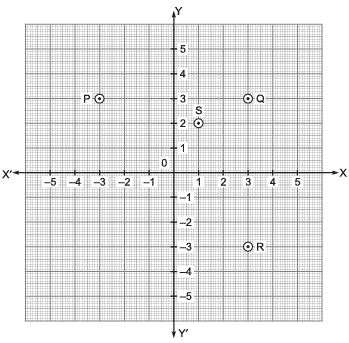Courses

# Very Short Answer Type Questions- Coordinate Geometry Class 9 Notes | EduRev

## Mathematics (Maths) Class 9

Created by: Full Circle

## Class 9 : Very Short Answer Type Questions- Coordinate Geometry Class 9 Notes | EduRev

The document Very Short Answer Type Questions- Coordinate Geometry Class 9 Notes | EduRev is a part of the Class 9 Course Mathematics (Maths) Class 9.
All you need of Class 9 at this link: Class 9

Question 1. A point (other than zero) is such that [The abscissa of the point] = [The ordinate of the point] In which quadrants can the point lie?
Solution: ∵ The coordinates have opposite signs in II and IV quadrants.
∴ the given point lies in I or III quadrant.

Question 2. A line ∠M is drawn parallel to X-axis at a distance of 5 units from it. On ∠M a point P is marked at a distances of 3 units from the Y-axis. What are the coordinates of P?
Solution: The line M can be above or below the x-axis
∴ co-ordiantes of P are (3, 5) or (–3, 5)

Question 3. Which of the following statements are true?
(i) The point (0, –4) lies on y-axis
(ii) The perpendicular distance of the point (5, 7) from the x-axis is 5.
(iii) Point (1, –1) and (–1, 1) lie in the same quadrant.
Solution: Only the statement (i) is true.

Question 4. What are the coordinates of origin?
Solution: (0, 0) are the coordinates of origin.

Question 5. What is the sign of y-coordinate below the x-axis?
Solution: It is always negative.

Question 6. What is the x-coordinate of a point on y-axis?
Solution: It is always ‘0’.

Question 7. In which quadrant the point (–2, 5) lies?
Solution: (–2, 5) lies in the II-quadrant.

Question 8. What is the sign of the y-coordinate of a point lying in the III-quadrant?
Solution: The y-coordinate of a point in III-quadrant is always negative.

Question 9. If the two points are A(–2, 7) and B(–3, 4) then what is (abscissa of A) – (abscissa of B)?
Solution: ∵ abscissa of A = – 2 and abscissa of B = – 3
∵ (– 2) – (– 3) = –2 + 3 =1

Question 10. If the y-coordinate of a point is zero, then where does this point lie?
Solution: It lies on the x-axis.

Question 11. What are the coordinates of a point lying on the y-axis at negative 3 units?
Solution: (0, –3)

Question 12. What are the coordinates of a point whose ordinate is 5 and lying on the y-axis.
Solution: (0, 5).

Question 13. In the following figure, which point has its abscissa as –3
Solution: The point p(–3, 3) has its abscissa as –3.Question 14. Name the collinear points in the above figure?
Solution: The points P, O and R are collinear.

Question 15. What is the distance of the point (3, – 4) from x-axis?
Solution: –4 units.

Question 16. The origin lies on which of the following? x-axis only, both axis, none of the axes
Solution: The origin lies on both the axes.

Question 17. The abscissa of a point is 5 and it lies on the x-axis. What are its coordinates?
Solution: (5, 0).

Question 18. A point lies on the x-axis as well as on the y-axis. What are its coordinates?
Solution: (0, 0)

Question 19. In which quadrants x and y coordinates have different sign

Question 20. In which quadrants x and y coordinate have same sign?

,

,

,

,

,

,

,

,

,

,

,

,

,

,

,

,

,

,

,

,

,

;